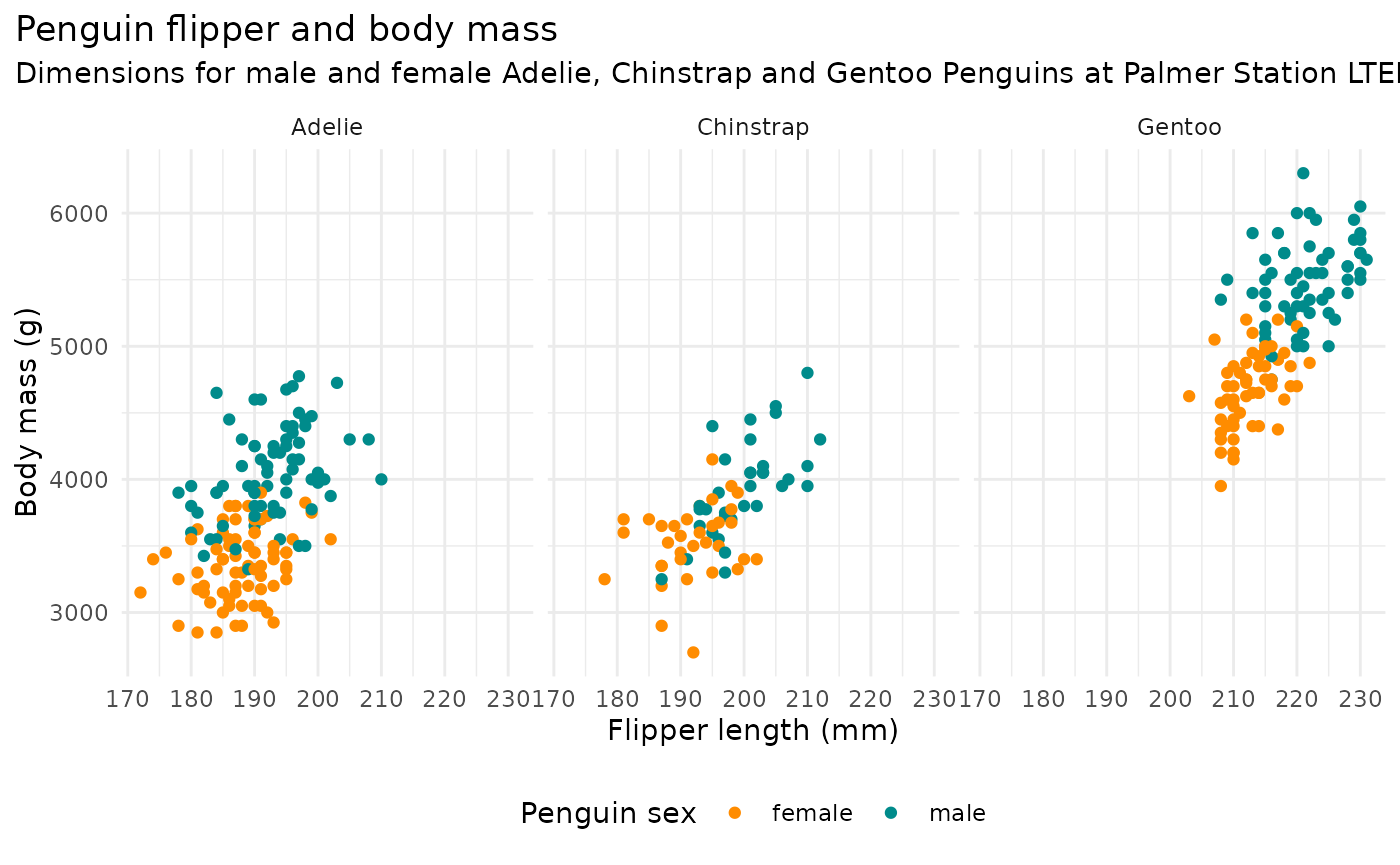library(palmerpenguins)
library(ggplot2)
ggplot2::theme_set(ggplot2::theme_minimal())

## Penguin mass vs. flipper length

mass_flipper <- ggplot(data = penguins,
aes(x = flipper_length_mm,
y = body_mass_g)) +
geom_point(aes(color = species,
shape = species),
size = 3,
alpha = 0.8) +
scale_color_manual(values = c("darkorange","purple","cyan4")) +
labs(title = "Penguin size, Palmer Station LTER",
subtitle = "Flipper length and body mass for Adelie, Chinstrap and Gentoo Penguins",
x = "Flipper length (mm)",
y = "Body mass (g)",
color = "Penguin species",
shape = "Penguin species") +
theme(legend.position = c(0.2, 0.7),
plot.title.position = "plot",
plot.caption = element_text(hjust = 0, face= "italic"),
plot.caption.position = "plot")

mass_flipper
#> Warning: Removed 2 rows containing missing values (geom_point).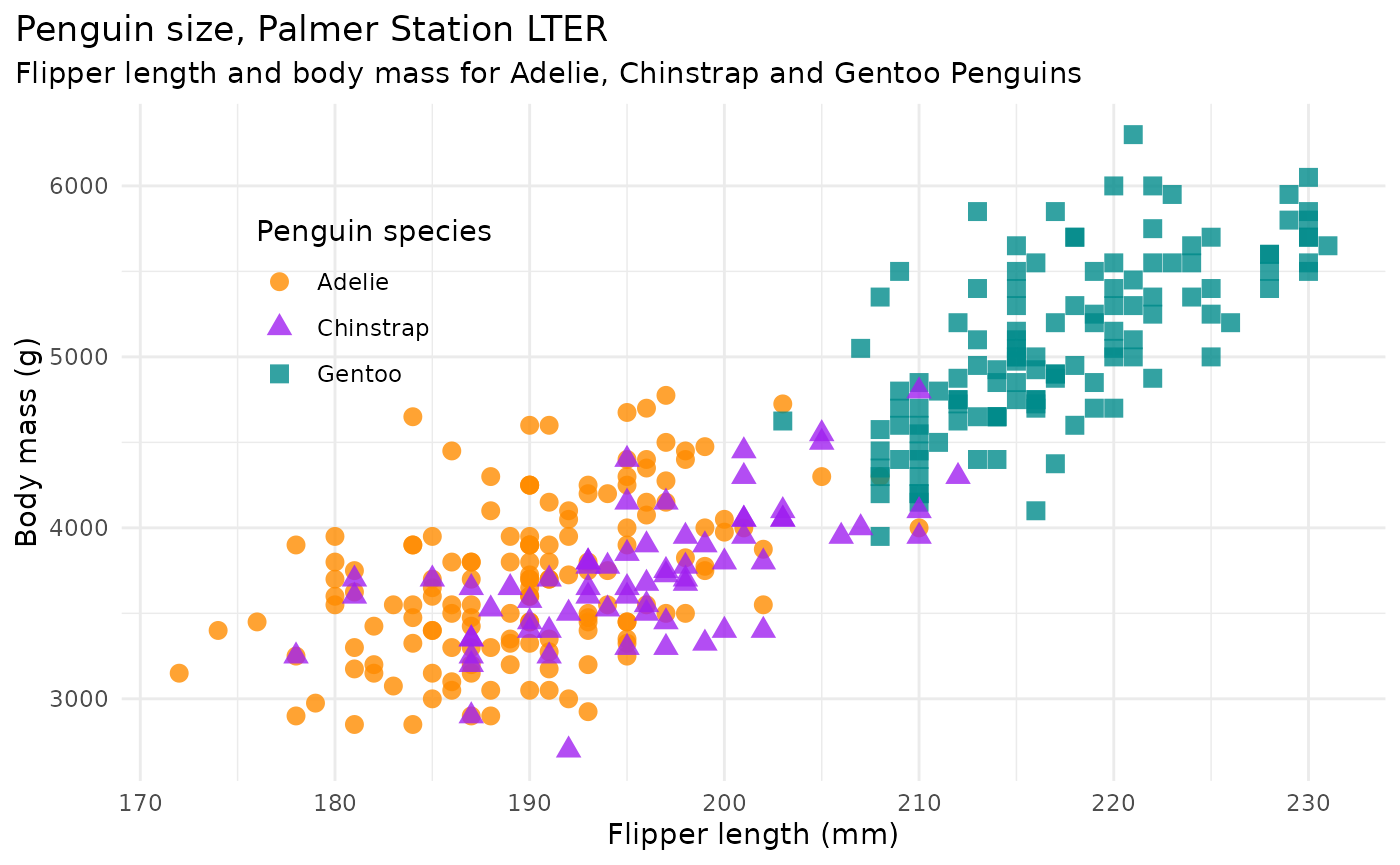## Flipper length vs. bill length

flipper_bill <- ggplot(data = penguins,
aes(x = flipper_length_mm,
y = bill_length_mm)) +
geom_point(aes(color = species,
shape = species),
size = 3,
alpha = 0.8) +
scale_color_manual(values = c("darkorange","purple","cyan4")) +
labs(title = "Flipper and bill length",
subtitle = "Dimensions for Adelie, Chinstrap and Gentoo Penguins at Palmer Station LTER",
x = "Flipper length (mm)",
y = "Bill length (mm)",
color = "Penguin species",
shape = "Penguin species") +
theme(legend.position = c(0.85, 0.15),
plot.title.position = "plot",
plot.caption = element_text(hjust = 0, face= "italic"),
plot.caption.position = "plot")

flipper_bill
#> Warning: Removed 2 rows containing missing values (geom_point).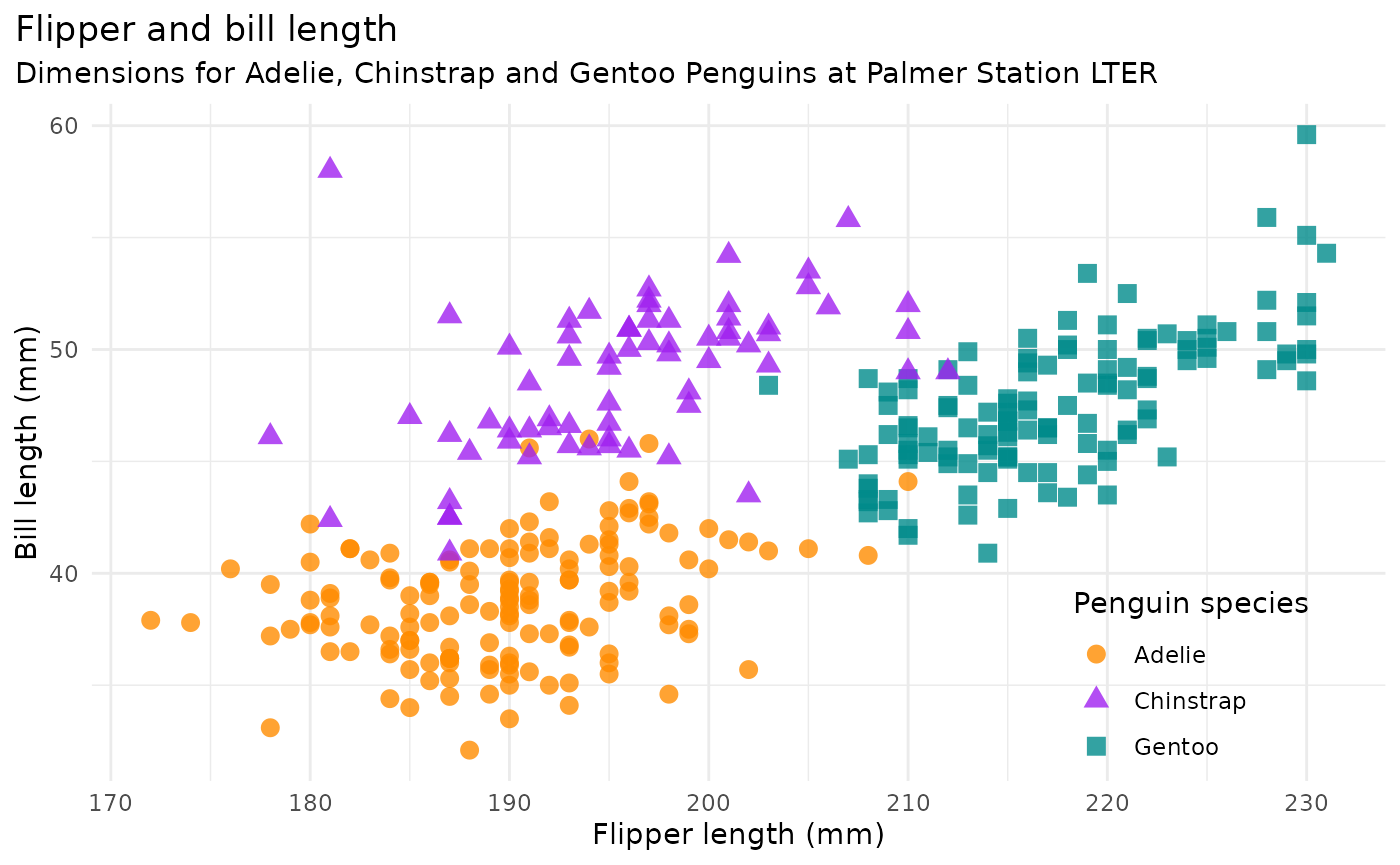## Bill length vs. depth

bill_len_dep <- ggplot(data = penguins,
aes(x = bill_length_mm,
y = bill_depth_mm,
group = species)) +
geom_point(aes(color = species,
shape = species),
size = 3,
alpha = 0.8) +
geom_smooth(method = "lm", se = FALSE, aes(color = species)) +
scale_color_manual(values = c("darkorange","purple","cyan4")) +
labs(title = "Penguin bill dimensions",
subtitle = "Bill length and depth for Adelie, Chinstrap and Gentoo Penguins at Palmer Station LTER",
x = "Bill length (mm)",
y = "Bill depth (mm)",
color = "Penguin species",
shape = "Penguin species") +
theme(legend.position = c(0.85, 0.15),
plot.title.position = "plot",
plot.caption = element_text(hjust = 0, face= "italic"),
plot.caption.position = "plot")

bill_len_dep
#> geom_smooth() using formula 'y ~ x'
#> Warning: Removed 2 rows containing non-finite values (stat_smooth).
#> Warning: Removed 2 rows containing missing values (geom_point).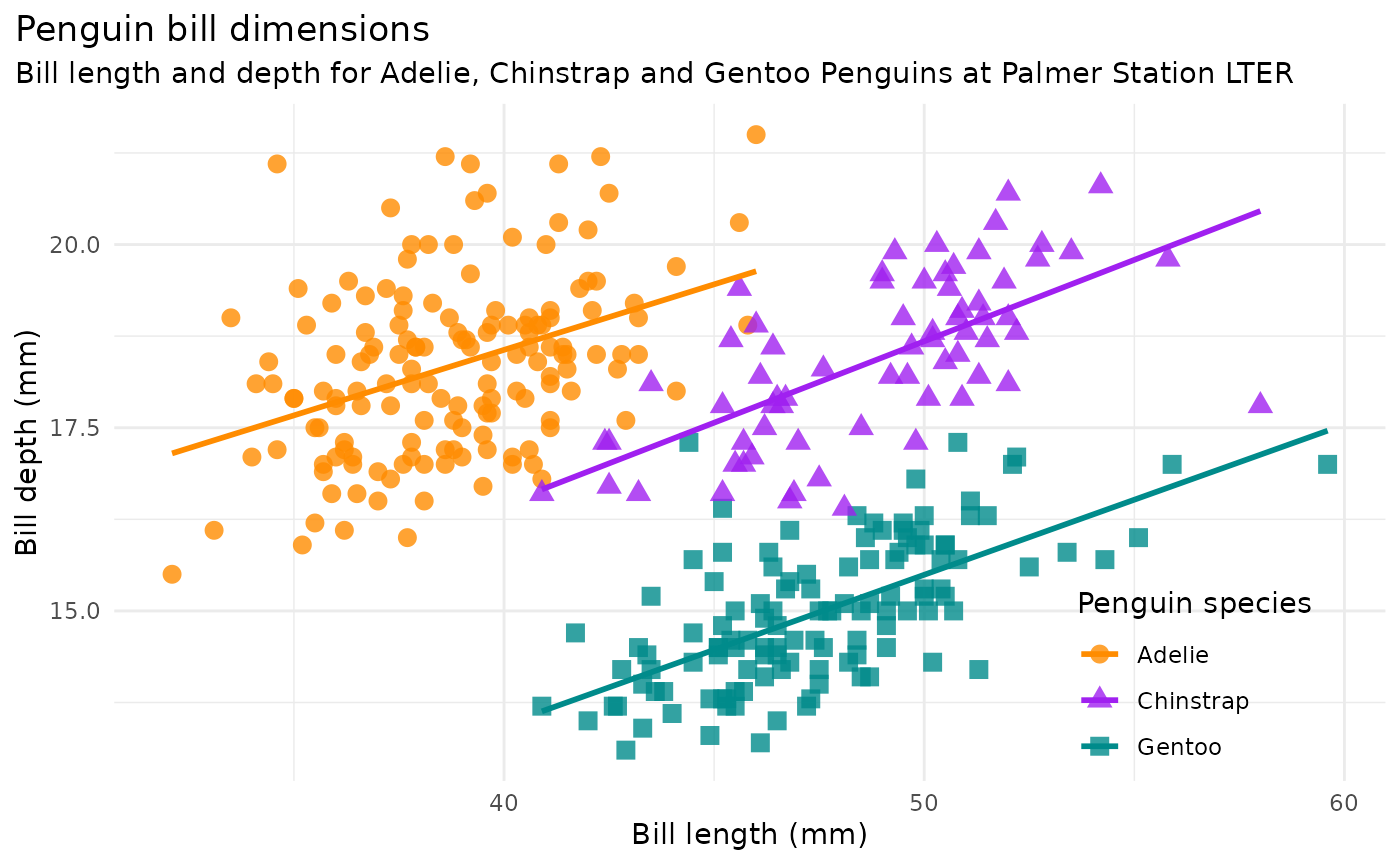## Simpson’s paradox, omitting species in graph above:

bill_no_species <- ggplot(data = penguins,
aes(x = bill_length_mm,
y = bill_depth_mm)) +
geom_point() +
scale_color_manual(values = c("darkorange","purple","cyan4")) +
labs(title = "Penguin bill dimensions (omit species)",
subtitle = "Palmer Station LTER",
x = "Bill length (mm)",
y = "Bill depth (mm)") +
theme(plot.title.position = "plot",
plot.caption = element_text(hjust = 0, face= "italic"),
plot.caption.position = "plot") +
geom_smooth(method = "lm", se = FALSE, color = "gray50")

bill_no_species
#> geom_smooth() using formula 'y ~ x'
#> Warning: Removed 2 rows containing non-finite values (stat_smooth).
#> Warning: Removed 2 rows containing missing values (geom_point).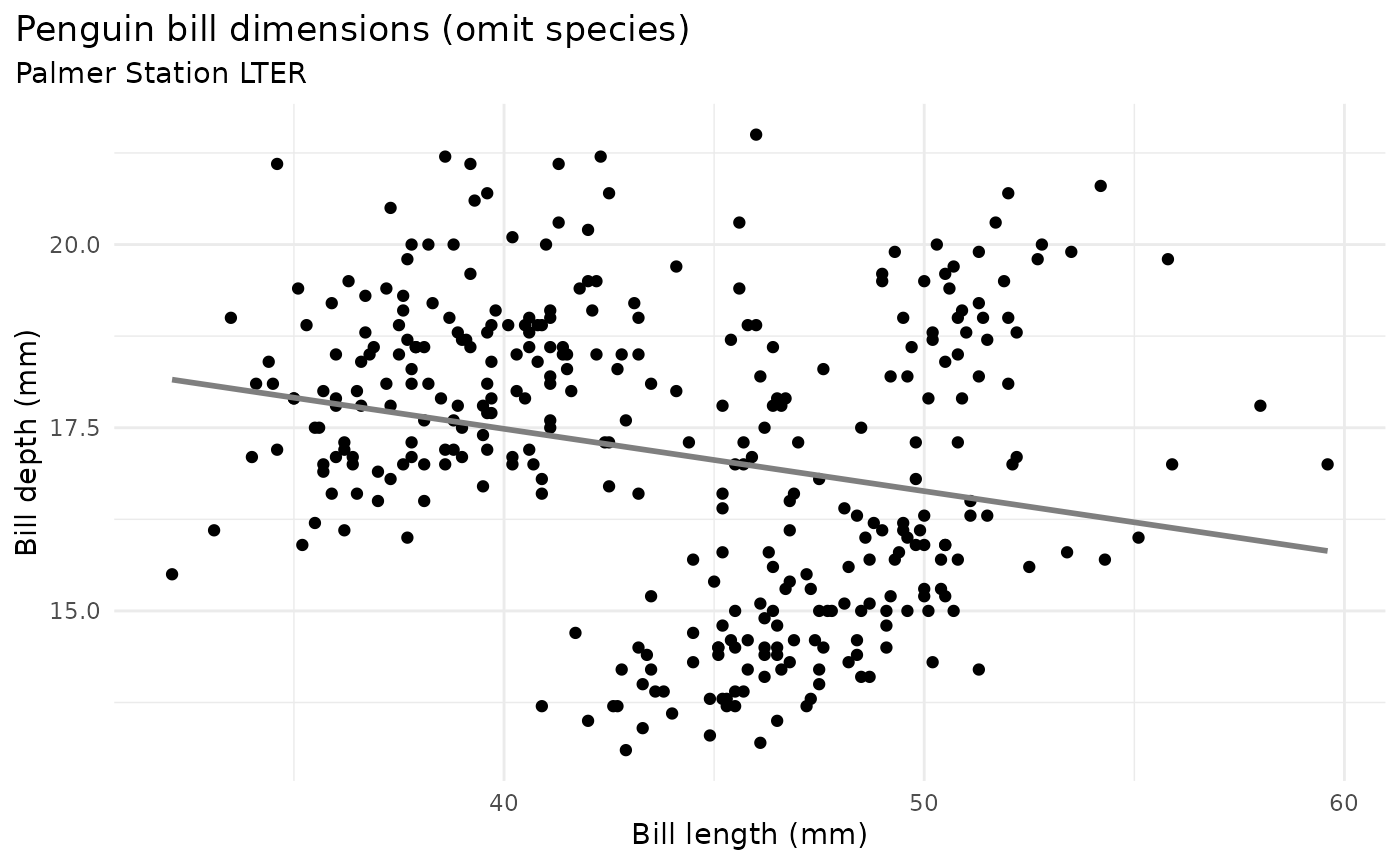## Some other graphs for brainstorming

There are a number of questions you could ask about differences between groups. Here are a couple histograms to consider.


flipper_hist <- ggplot(data = penguins, aes(x = flipper_length_mm)) +
geom_histogram(aes(fill = species),
alpha = 0.5,
position = "identity") +
scale_fill_manual(values = c("darkorange","purple","cyan4")) +
labs(x = "Flipper length (mm)",
y = "Frequency",
title = "Penguin flipper lengths")

flipper_hist
#> stat_bin() using bins = 30. Pick better value with binwidth.
#> Warning: Removed 2 rows containing non-finite values (stat_bin).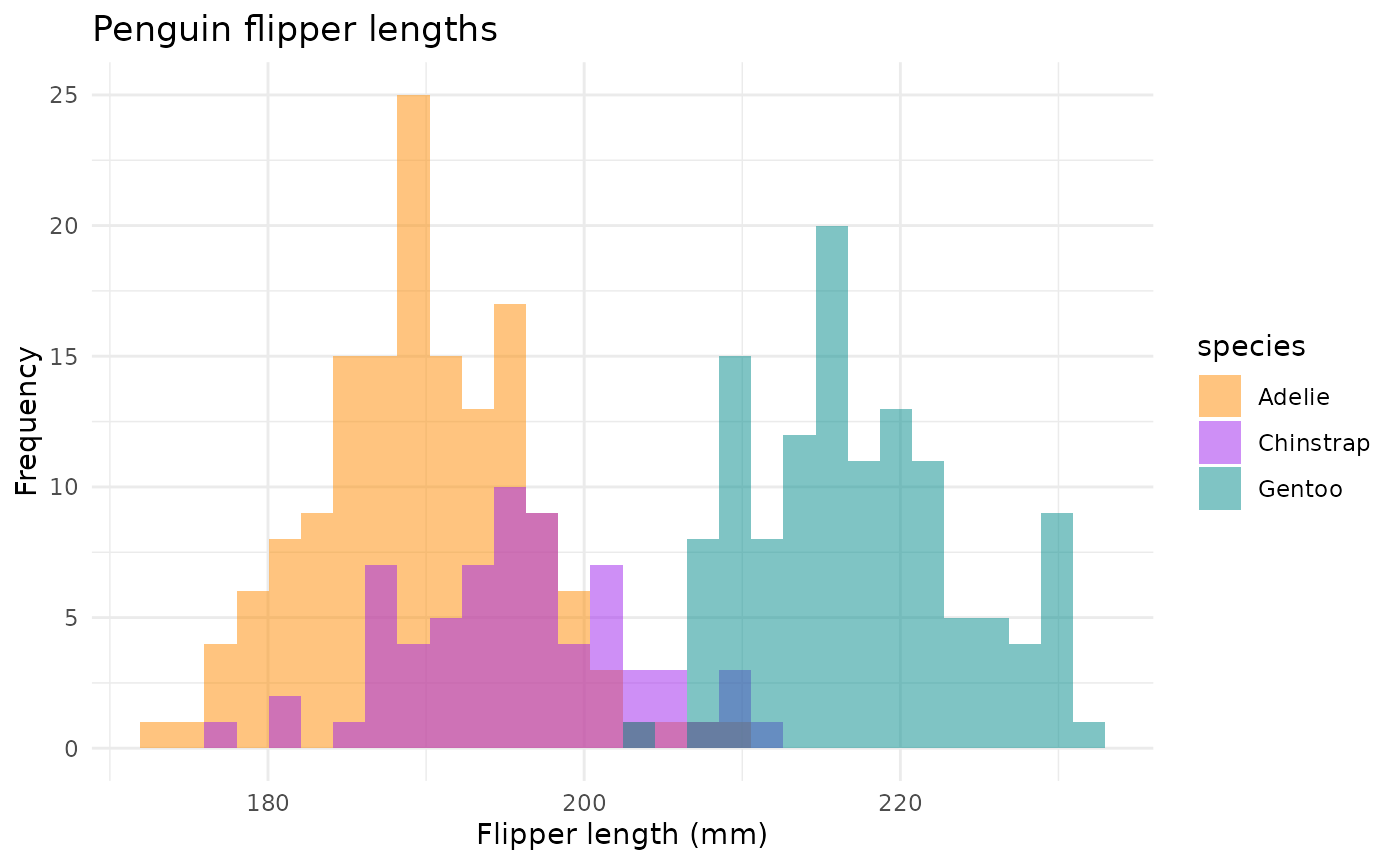Flipper lengths boxplots + jitter:

flipper_box <- ggplot(data = penguins, aes(x = species, y = flipper_length_mm)) +
geom_boxplot(aes(color = species), width = 0.3, show.legend = FALSE) +
geom_jitter(aes(color = species), alpha = 0.5, show.legend = FALSE, position = position_jitter(width = 0.2, seed = 0)) +
scale_color_manual(values = c("darkorange","purple","cyan4")) +
labs(x = "Species",
y = "Flipper length (mm)")

flipper_box
#> Warning: Removed 2 rows containing non-finite values (stat_boxplot).
#> Warning: Removed 2 rows containing missing values (geom_point).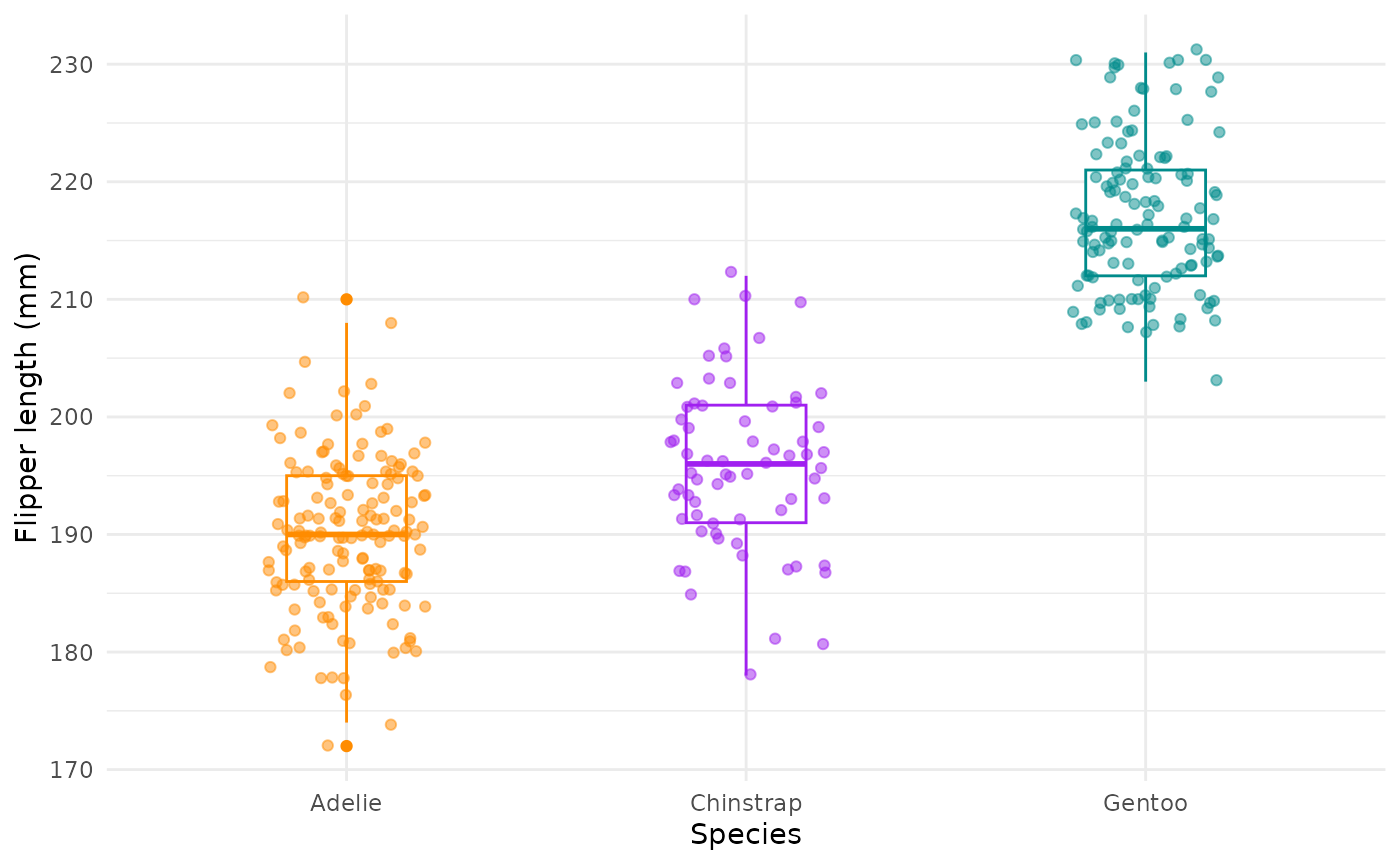Body mass histograms:


mass_hist <- ggplot(data = penguins, aes(x = body_mass_g)) +
geom_histogram(aes(fill = species),
alpha = 0.5,
position = "identity") +
scale_fill_manual(values = c("darkorange","purple","cyan4")) +
labs(x = "Body mass (g)",
y = "Frequency",
title = "Penguin body mass")

mass_hist
#> stat_bin() using bins = 30. Pick better value with binwidth.
#> Warning: Removed 2 rows containing non-finite values (stat_bin).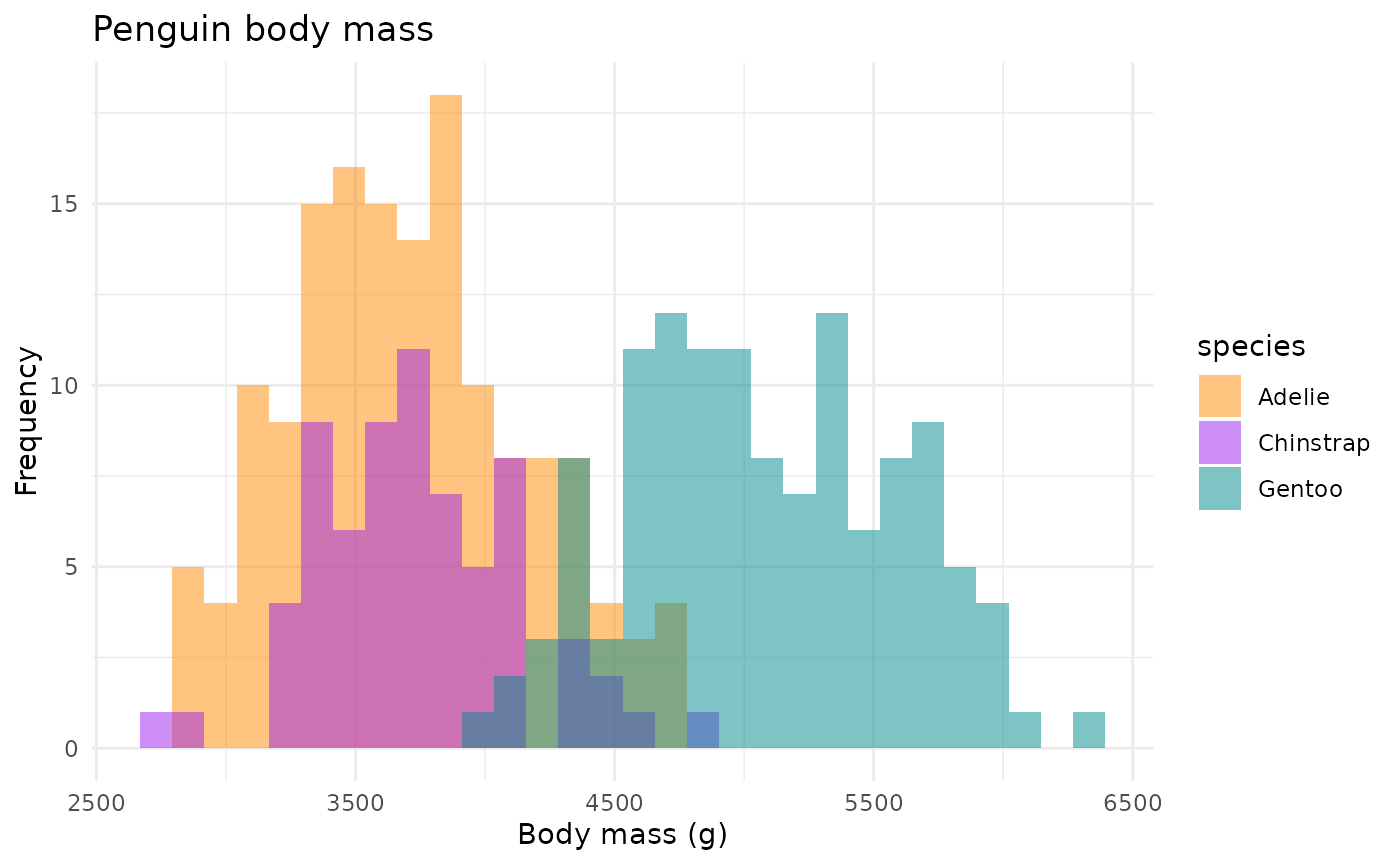## Facets

Include penguin sex:


ggplot(penguins, aes(x = flipper_length_mm,
y = body_mass_g)) +
geom_point(aes(color = sex)) +
scale_color_manual(values = c("darkorange","cyan4"), na.translate = FALSE) +
labs(title = "Penguin flipper and body mass",
subtitle = "Dimensions for male and female Adelie, Chinstrap and Gentoo Penguins at Palmer Station LTER",
x = "Flipper length (mm)",
y = "Body mass (g)",
color = "Penguin sex") +
theme(legend.position = "bottom",
plot.title.position = "plot",
plot.caption = element_text(hjust = 0, face= "italic"),
plot.caption.position = "plot") +
facet_wrap(~species)
#> Warning: Removed 11 rows containing missing values (geom_point).